Next: Average number of different Up: Modular mappings Previous: Square roots of integers

## Algebraic numbers

An algebraic number is a value which satisfies a certain polynomial relation. E.g. Let

p(x) = x3-2x+5

then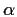such that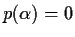is an algebraic number. Square roots are a special case of algebraic numbers, when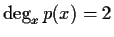.

If the polynomial has coefficients which are not numerical constants, thenis called an algebraic function.

To obtain signatures of algebraic numbers we need to find values of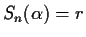such that Sn( p(r) ) = 0. This can be computed by finding all the different linear factors of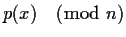. This is simpler than factorization, it can be obtained from the first step of the Cantor-Zassenhaus factorization algorithm.

The same problems that we have with square roots will show with algebraic numbers:

(a) p(x) may have no linear factors, hence no roots mod n, hence no possible value for r.

(b) there may be more than one different linear factor and choosing is a problem.

For algebraic numbers of degree 3 or larger it is sometimes possible to find an n which produces a factorization which has a single linear factor (possibly with some multiplicity) and hence a single root. This is extremely desirable, and justifies trying several values of n to see if this is possible. When we have a unique root we can consider it as the signature for the algebraic number. In this case, since factorization requires polynomial time in the degree of p(x), the signature can be computed in linear time on the size plus polynomial time on the degrees of its algebraic numbers.

If the polynomial does not have any linear factors, we do not have any choice other than selecting a new nand trying again. For random polynomials and for large values of n, 1/2+O(1/n) of all polynomials of degree 3 have a single root, 1/3+O(1/n) for degree 4 and 3/8+O(1/n) for degree 5.Next: Average number of different Up: Modular mappings Previous: Square roots of integers
Gaston Gonnet
1999-07-04